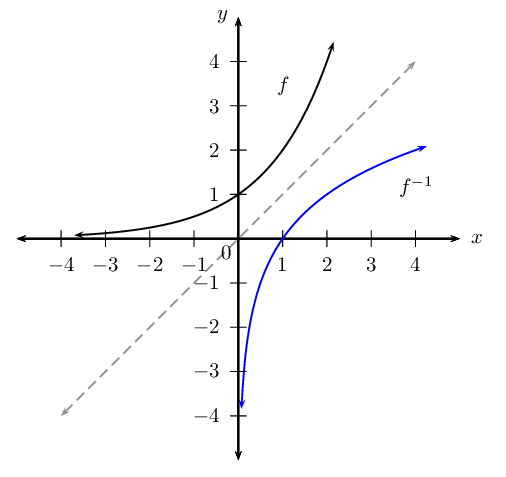Home Practice
For learners and parents For teachers and schools
Textbooks
Full catalogue
Pricing SupportLog in

We think you are located in United States. Is this correct?

# 2.3 Inverse functions

## 2.3 Inverse functions (EMCF8)

An inverse function is a function which does the “reverse” of a given function. More formally, if $$f$$ is a function with domain $$X$$, then $${f}^{-1}$$ is its inverse function if and only if $${f}^{-1}\left(f\left(x\right)\right)=x$$ for every $$x \in X$$.

\begin{align*} & \\ y = f(x) &: \text{indicates a function} \\ & \\ {y}_{1} = f\left({x}_{1}\right) &: \text{indicates we must substitute a specific } x_{1} \text{ value} \\ & \quad \text{into the function to get the corresponding } y_{1}\text{ value} \\ & \\ {f}^{-1}\left(y\right) = x &: \text{indicates the inverse function} \\ & \\ {f}^{-1}\left(y_{1}\right) = {x}_{1} &: \text{indicates we must substitute a specific } y_{1} \text{ value} \\ & \quad \text{into the inverse to return the specific } x_{1}\text{ value} \end{align*}

A function must be a one-to-one relation if its inverse is to be a function. If a function $$f$$ has an inverse function $$f^{-1}$$, then $$f$$ is said to be invertible.

Given the function $$f(x)$$, we determine the inverse $$f^{-1}(x)$$ by:

• interchanging $$x$$ and $$y$$ in the equation;
• making $$y$$ the subject of the equation;
• expressing the new equation in function notation.

Note: if the inverse is not a function then it cannot be written in function notation. For example, the inverse of $$f(x) = 3x^2$$ cannot be written as $$f^{-1}(x) = \pm \sqrt{\frac{1}{3}x}$$ as it is not a function. We write the inverse as $$y = \pm \sqrt{\frac{1}{3}x}$$ and conclude that $$f$$ is not invertible.

If we represent the function $$f$$ and the inverse function $${f}^{-1}$$ graphically, the two graphs are reflected about the line $$y=x$$. Any point on the line $$y = x$$ has $$x$$- and $$y$$-coordinates with the same numerical value, for example $$(-3;-3)$$ and $$\left( \frac{4}{5}; \frac{4}{5} \right)$$. Therefore interchanging the $$x$$- and $$y$$-values makes no difference.This diagram shows an exponential function (black graph) and its inverse (blue graph) reflected about the line $$y = x$$ (grey line).

Important: for $${f}^{-1}$$, the superscript $$-\text{1}$$ is not an exponent. It is the notation for indicating the inverse of a function. Do not confuse this with exponents, such as $$\left( \frac{1}{2} \right)^{-1}$$ or $$3 + x^{-1}$$.

Be careful not to confuse the inverse of a function and the reciprocal of a function:

 Inverse Reciprocal $$f^{-1}(x)$$ $$[f(x)]^{-1} = \frac{1}{f(x)}$$ $$f(x)$$ and $$f^{-1}(x)$$ symmetrical about $$y=x$$ $$f(x) \times \frac{1}{f(x)} = 1$$ Example: $$\qquad \qquad \qquad \qquad \qquad \qquad$$ Example: $$\qquad \qquad \qquad \qquad \qquad$$ $$g(x) = 5x \therefore g^{-1}(x)= \frac{x}{5}$$ $$g(x) = 5x \therefore \frac{1}{g(x)} = \frac{1}{5x}$$
temp text